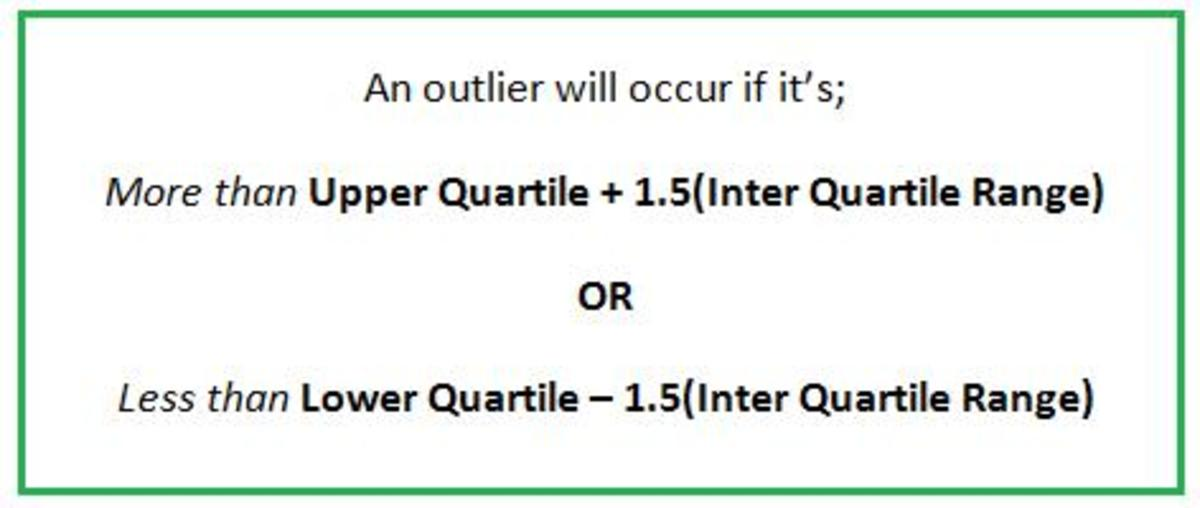Updated date:

# Box Plots With Outliers. What Is an Outlier and How to Find These Extreme Points.

Author:Outliers are extreme values that lie within a set of data. If you had to draw a box and whisker diagram then the outliers should be marked on with a cross.

In order to identify an outlier first find the Lower Quartile, Upper Quartile and Inter Quartile Range.

Now an outlier will occur if it’s;

More than Upper Quartile + 1.5(Inter Quartile Range)

OR

Less than Lower Quartile – 1.5(Inter Quartile Range)

Other scale factors can be used instead of 1.5 but 1.5 is the most commonly used. The exam question will tell you if it’s any different.

Let’s take a look at an example:

Example 1

A class of 15 students took a Science exam. Here are the results of the class:

8%, 9%, 40%, 52%, 53%, 60%, 62%, 62%, 64%, 68%, 70%, 70%, 71%,71%, 98%

Work out the lower quartile, upper quartile and inter quartile range. Also identify any outliers that occur within the test scores:

First calculate the lower quartile:

¼ of 15 = 3.75 = 4th person (always round upwards if it comes out as a decimal)

So the lower quartile is 52% as this is the 4th persons score.

Next work out the upper quartile:

¾ of 15 = 11.25 = 12th person (again round upwards as the answer came out as a decimal)

So the upper quartile is 70% as the 12th highest score in the data was 70%

Since you have the upper quartile and lower quartile, find the difference of these to give you the inter quartile range, 70 – 52 = 18%

So our three values that you need to work out the outliers are:

Lower Quartile = 52%

Upper Quartile = 70%

Inter Quartile Range = 18%

First let’s work out the outliers that occur at the upper end of the data set:

Upper Quartile + 1.5(Inter Quartile Range)

= 70 + 1.5x18 = 97%

Any values which are more than 97% will be outliers. So the person who scored 98% is an outlier.

Next work out the outliers that occur at the lower end of the data set:

Lower Quartile – 1.5(Inter Quartile Range)

= 52 – 1.5 x 18 = 25%

So any values which are less than 25% are also outliers. So looking back at the data there are two outliers that are less than 25% which are 8% and 9%.

So altogether there are 3 outliers in this data set; 8%,9% and 98%.

Summary

So remember that outlier are extreme values. They are either more than Upper Quartile + 1.5(Inter Quartile Range) or less than Lower Quartile – 1.5(Inter Quartile Range).